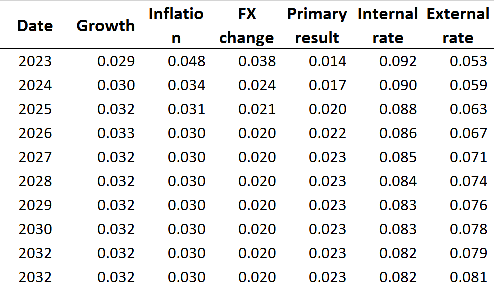# Debt Sustainability Analysis - Costa Rica Dec 2022

Our Debt Sustainability Model uses the standard debt dynamic equation to generate forecasts for the Debt/GDP ratio throughout the next 10 years. Specifically, we use the following equation:

$d_{t+1} =d_{t}\biggl[(1-\alpha)\biggl(\frac{1+i_{dt}}{(1+g_t)(1+\pi_t)}\biggl) +(\alpha)\biggl(\frac{(1+\Delta\epsilon_t)(1+i_{ft})}{(1+g_t)(1+\pi_t)}\biggl)\biggl] + pd_t$

In which:

$d_{t+1}$ is the ratio Debt/GDP for the t+1 period;

$\alpha$ is the share of foreign debt in relation to total debt;

$g_t$ is the real growth of GDP at the period t;

$\pi_t$ is the variation of the GDP deflator for the period t;

$\Delta\epsilon_t$ is the devaluation of the exchange rate between the local currency to the US dollar during the period t;

$i_{dt}$ is the implicit interest rate for the domestic debt, it is calculated as the ratio between the interest paid for the internal debt during the period t and the internal debt in the period t-1;

$i_{ft}$ is the implicit interest rate for the foreign debt, it is calculated as the ratio between the interest paid for the internal debt during the period t and the internal debt in the period t-1;

$pd_t$ is the primary deficit of the government during the period t.

We account for uncertainty by applying random shocks for each variable. These shocks are constructed by simulating a Multivariate Normal Distribution that uses as input the historical covariance matrix between those variables and their forecasts for the next 10 years. We simulate 2000 shocks for each year and compute the quantiles of the Debt/GDP forecasts according to our DSA equation.

## Model Results. Debt / GDP Dynamic## Baseline Assumption

The parameters of this simulation can be changed updated and running this pipelineThe interest rate forecast is estimated using a starting average implied rate of 9.3% and 4.6% respectively for internal and external. 20% of the debt is renewed at the new marginal rate of 7.2% (3.7% USD 5y + 350bp) for external and 8% for internal

## Scenarios and sensibility Analysis

The chart below reports the results of the simulation of shocks over a single variable, while the other variables are kept at their baseline levels## Annex: Model inputsWritten by

Luciano Cohan

Co-Fundador de Alphacast. Ex Subsecretario de Programación Macroeconómica. Data Science. Creando una plataforma para el trabajo colaborativo en economías

## Related insights

$d_{t+1} =d_{t}\biggl[(1-\alpha)\biggl(\frac{1+i_{dt}}{(1+g_t)(1+\pi_t)}\biggl) +(\alpha)\biggl(\frac{(1+\Delta\epsilon_t)(1+i_{ft})}{(1+g_t)(1+\pi_t)}\biggl)\biggl] + pd_t$
$d_{t+1}$ is the ratio Debt/GDP for the t+1 period;
$\alpha$ is the share of